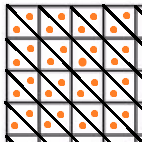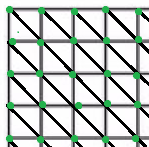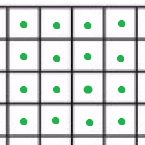# Get nearest vertex instead of node?

I’m hoping to implement a form of snapping to my navmesh graph based on cursor input. I currently have it “working” by using this code:

``````closestNode = (Vector3)AstarPath.active.graphs.GetNearest(hitInfo.point).node.position;
``````

While it technically works, they are the centers of triangles instead of an actual grid:The above orange dots don’t make for very good grid snapping!

I was hoping that instead of getting the nearest node that I could get the nearest vertex.Or get the nearest quad instead of triangle:Is there a way to access this graph data so I can get a more reliable grid?

Hi

Sorry, this is not possible. You can get the nearest node and then access its vertices and manually find the closest one of those. That’s not quite equivalent, but it might be good enough?

The graph doesn’t know about quads at all.

Certainly worth a shot! What would be your recommended approach to access a node’s vertices? I tried looking in the docs for a way but came up empty.

You can use TriangleMeshNode.GetVertex

Thanks so much, we got it working! Here’s our code in case someone else in the future needs it:

``````TriangleMeshNode closestNodeTriangle;
Int3 vertA;
Int3 vertB;
Int3 vertC;
Vector3[] triangleArray = new Vector3;
Vector3 bestSnap;
float distSqr;
float closestDistSqr;
Vector3 directionToTarget;

if (Physics.Raycast(ray, out hitInfo, 100, mask))
{
// Get the node triangle into an array
closestNodeTriangle = AstarPath.active.graphs.GetNearest(hitInfo.point).node as
TriangleMeshNode;
closestNodeTriangle.GetVertices(out vertA, out vertB, out vertC);
// Yes we have to explicitly cast these because the GetVertices method refuses to cooperate
triangleArray = (Vector3)vertA;
triangleArray = (Vector3)vertB;
triangleArray = (Vector3)vertC;
closestDistSqr = Mathf.Infinity;
// Iterate through each of the 3 vertices and pick the closest one to the cursor
for (int i = 0; i < 3; i++)
{
directionToTarget = triangleArray[i] - hitInfo.point;
distSqr = directionToTarget.sqrMagnitude;
if (distSqr < closestDistSqr)
{
closestDistSqr = distSqr;
bestSnap = triangleArray[i];
}
// Assign the object to the closest vertex
tower.transform.position = bestSnap;
}
}
``````
1 Like